# Inductance

## DC Electric Circuits

• #### Question 1

One of the fundamental principles of calculus is a process called integration. This principle is important to understand because it is manifested in the behavior of inductance. Thankfully, there are more familiar physical systems which also manifest the process of integration, making it easier to comprehend.

If we introduce a constant flow of water into a cylindrical tank with water, the water level inside that tank will rise at a constant rate over time: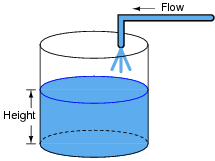In calculus terms, we would say that the tank integrates water flow into water height. That is, one quantity (flow) dictates the rate-of-change over time of another quantity (height).

Like the water tank, electrical inductance also exhibits the phenomenon of integration with respect to time. Which electrical quantity (voltage or current) dictates the rate-of-change over time of which other quantity (voltage or current) in an inductance? Or, to re-phrase the question, which quantity (voltage or current), when maintained at a constant value, results in which other quantity (current or voltage) steadily ramping either up or down over time?

• #### Question 2

Suppose a mass is connected to a winch by means of a cable, and a person turns the winch drum to raise the mass off the ground: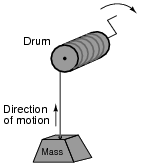A physicist would likely look at this scenario as an example of energy exchange: the person turning the drum is expending energy, which in turn is being stored in the mass in potential form.

Suppose now that the person stops turning the drum and instead engages a brake mechanism on the drum so that it reverses rotation and slowly allows the mass to return to ground level. Once again, a physicist would view this scenario as an exchange of energy: the mass is now releasing energy, while the brake mechanism is converting that released energy into heat: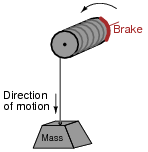In each of the above scenarios, draw arrows depicting directions of two forces: the force that the mass exerts on the drum, and the force that the drum exerts on the mass. Compare these force directions with the direction of motion in each scenario, and explain how these directions relate to the mass and drum alternately acting as energy source and energy load.

• #### Question 3

Draw the direction of current in this circuit, and also identify the polarity of the voltage across the battery and across the resistor. Then, compare the battery’s polarity with the direction of current through it, and the resistor’s polarity with the direction of current through it.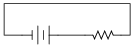What do you notice about the relationship between voltage polarity and current direction for these two different types of components? Identify the fundamental distinction between these two components that causes them to behave differently.

• #### Question 4

Draw the pattern of the magnetic field produced by electric current through a straight wire and through a wire coil: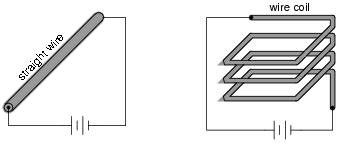Explain your answer using either the right-hand rule (conventional flow) or the left-hand rule (electron flow).

• #### Question 5

As an electric current is passed through a coil of wire, it creates a magnetic field. If the magnitude of this current changes over time, so will the strength of the magnetic field.

We also know that a magnetic field flux that changes over time will induce a voltage along the length of a wire coil. Explain how the complementary principles of electromagnetism and electromagnetic induction manifest themselves simultaneously in the same wire coil to produce self-induction.

Also, explain how Lenz’s Law relates to the polarity of the coil’s self-induced voltage.

• #### Question 6

In a simple resistor circuit, the current may be calculated by dividing applied voltage by resistance:Although an analysis of this circuit probably seems trivial to you, I would like to encourage you to look at what is happening here from a fresh perspective. An important principle observed many times in the study of physics is that of equilibrium, where quantities naturally “seek” a state of balance. The balance sought by this simple circuit is equality of voltage: the voltage across the resistor must settle at the same value as the voltage output by the source: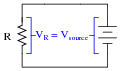If the resistor is viewed as a source of voltage seeking equilibrium with the voltage source, then current must converge at whatever value necessary to generate the necessary balancing voltage across the resistor, according to Ohm’s Law (V = IR). In other words, the resistor’s current achieves whatever magnitude it has to in order to generate a voltage drop equal to the voltage of the source.

This may seem like a strange way of analyzing such a simple circuit, with the resistor “seeking” to generate a voltage drop equal to the source, and current “magically” assuming whatever value it must to achieve that voltage equilibrium, but it is helpful in understanding other types of circuit elements.

For example, here we have a source of DC voltage connected to a large coil of wire through a switch. Assume that the wire coil has negligible resistance (0 Ω):Like the resistor circuit, the coil will “seek” to achieve voltage equilibrium with the voltage source once the switch is closed. However, we know that the voltage induced in a coil is not directly proportional to current as it is with a resistor - instead, a coil’s voltage drop is proportional to the rate of change of magnetic flux over time as described by Faraday’s Law of electromagnetic induction:

 vcoil = N d φ dt

Where,

vcoil = Instantaneous induced voltage, in volts

N = Number of turns in wire coil

$$\frac{dφ}{dt}$$ = Instantaneous rate of change of magnetic flux, in webers per second

Assuming a linear relationship between coil current and magnetic flux (i.e. φ doubles when i doubles), describe this simple circuit’s current over time after the switch closes.

• #### Question 7

Inductance is a very important property in many types of electric circuits. Define what “inductance” is, and what causes it.

• #### Question 8

If the number of turns of wire in an electromagnet coil is tripled, what happens to the magnitude of the magnetic flux (Φ) generated by it, assuming that none of the other variables change (current through the coil, reluctance of magnetic circuit, etc.)?

If the number of turns of wire in an inductor is tripled, what happens to the magnitude of the induced voltage for a given rate of magnetic flux change over time $$\frac{dφ}{dt}$$?

If the number of turns of wire in an inductor is tripled, what happens to the magnitude of its inductance, measured in Henrys? Explain your answer.

• #### Question 9

The amount of inductance inherent in a wire coil may be calculated by the following equation:

 L = N2 A μ l

Where,

L = Inductance in Henrys

N = Number of wire “turns” wrapped around the core

μ = Permeability of core material (absolute, not relative)

A = Core area, in square meters

l = Length of core, in meters

Calculate how many turns of wire must be wrapped around a hollow, non-magnetic (air) core 2 cm in diameter and 10 cm in length in order to create an inductance of 22 mH. You may use the permeability of free space (μ0) for the μ value of the air core.

Next, calculate the required number of turns to produce the same inductance with a solid iron core of the same dimensions, assuming that the iron has a relative permeability (μr) of 4000.

Finally, knowing that the formula for the area of a circle is πr2, re-write the inductance equation so as to accept a value for inductor radius rather than inductor area. In other words, substitute radius (r) for area (A) in this equation in such a way that it still provides an accurate figure for inductance.

• #### Question 10

Suppose you wished to build a component with no other purpose than to provide inductance in an electric circuit (an inductor). How might you design such a device to perform this function, and how could you maximize its inductance?

• #### Question 11

Magnetic fields, like all fields, have two fundamental measures: field force and field flux. In an inductor, which of these field quantities is directly related to current through the wire coil, and which is directly related to the amount of energy stored?

Based on this relationship, which magnetic field quantity changes when a bar of iron is brought closer to a wire coil, connected to a source of constant current?• #### Question 12

Suppose an inductor is connected directly to an adjustable-current source, and the current of that source is steadily increased over time. We know that an increasing current through an inductor will produce a magnetic field of increasing strength. Does this increase in magnetic field constitute an accumulation of energy in the inductor, or a release of energy from the inductor? In this scenario, does the inductor act as a load or as a source of electrical energy?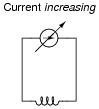Now, suppose the adjustable current source is steadily decreased over time. We know this will result in a magnetic field of decreasing strength in the inductor. Does this decrease in magnetic field constitute an accumulation of energy in the inductor, or a release of energy from the inductor? In this scenario, does the inductor act as a load or as a source of electrical energy?For each of these scenarios, label the inductor’s voltage drop polarity.

• #### Question 13

Ohm’s Law tells us that the amount of voltage dropped by a fixed resistance may be calculated as such:

 E = IR

However, the relationship between voltage and current for a fixed inductance is quite different. The “Ohm’s Law” formula for an inductor is as such:

 e = L di dt

What significance is there in the use of lower-case variables for current (i) and voltage (e)? Also, what does the expression [di/dt] mean? Note: in case you think that the d’s are variables, and should cancel out in this fraction, think again: this is no ordinary quotient! The d letters represent a calculus concept known as a differential, and a quotient of two d terms is called a derivative.

• #### Question 14

Complete this statement by substituting the correct electrical variables (voltage, current, resistance, inductance):

Inductors oppose changes in (fill-in-the-blank), reacting to such changes by producing a (fill-in-the-blank).
• #### Question 15

Many years ago, I decided to experiment with electromagnetism by making an electromagnet out of a spool of wire. I placed a steel bolt through the center of the spool so as to have a core of high permeability, and passed current from a battery through the wire to make a magnetic field. Not having any “jumper” wires, I held the wire ends of the spool in contact with the 9-volt battery terminals, one in each hand.

The electromagnet worked just fine, and I was able to move some steel paperclips with the magnetic field generated by it. However, when I broke the circuit by releasing one of the wire ends from the battery terminal it was touching, I received a small electric shock! Shown here is a schematic diagram of me, in the circuit: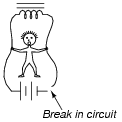At the time, I didn’t understand how inductance worked. I only understood how to make magnetism with electricity, but I didn’t realize a coil of wire could generate (high voltage!) electricity from its own magnetic field. I did know, however, that the 9 volts output by the battery was much too weak to shock me (yes, I touched the battery terminals directly to verify this fact), so something in the circuit must have generated a voltage greater than 9 volts.

If you had been there to explain what just happened to me, what would you say?

• #### Question 16

Components soldered into printed circuit boards often possess “stray” inductance, also known as parasitic inductance. Observe this resistor, soldered in to a circuit board: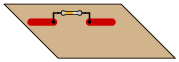Where does the parasitic inductance come from? What is it about a resistor, mounted to a circuit board, that creates a (very) small amount of inductance? How is it possible to minimize this inductance, in case it is detrimental to the circuit’s operation?

• #### Question 17

Many precision resistors utilize a wire-wound construction, where the resistance is determined by the type and length of wire wrapped around a spool. This form of construction allows for high precision of resistance, with low temperature sensitivity if certain metal alloys are used for the wire.

Unfortunately, though, wrapping wire around a spool forms a coil, which will naturally possess a significant amount of inductance. This is generally undesirable, as we would like to have resistors possessing only resistance, with no “parasitic” properties.

There is, however, a special way in which a wire coil may be wound so as to have almost no inductance. This method is called bifilar winding, and it is common in wire-wound resistor construction. Describe how bifilar winding works, and why it eliminates parasitic inductance.

• #### Question 18

Digital logic circuits, which comprise the inner workings of computers, are essentially nothing more than arrays of switches made from semiconductor components called transistors. As switches, these circuits have but two states: on and off, which represent the binary states of 1 and 0, respectively.

The faster these switch circuits are able to change state, the faster the computer can perform arithmetic and do all the other tasks computers do. To this end, computer engineers keep pushing the limits of transistor circuit design to achieve faster and faster switching rates.

This race for speed causes problems for the power supply circuitry of computers, though, because of the current “surges” (technically called transients) created in the conductors carrying power from the supply to the logic circuits. The faster these logic circuits change state, the greater the [di/dt] rates-of-change exist in the conductors carrying current to power them. Significant voltage drops can occur along the length of these conductors due to their parasitic inductance:Suppose a logic gate circuit creates transient currents of 175 amps per nanosecond (175 A/ns) when switching from the “off” state to the ön” state. If the total inductance of the power supply conductors is 10 picohenrys (9.5 pH), and the power supply voltage is 5 volts DC, how much voltage remains at the power terminals of the logic gate during one of these “surges”?

• #### Question 19

Electrical inductance has a close mechanical analogy: inertia. Explain what mechanical “inertia” is, and how the quantities of velocity and force applied to an object with mass are respectively analogous to current and voltage applied to an inductance.

• #### Question 20

Inductors store energy in the form of a magnetic field. We may calculate the energy stored in an inductance by integrating the product of inductor voltage and inductor current (P = IV) over time, since we know that power is the rate at which work (W) is done, and the amount of work done to an inductor taking it from zero current to some non-zero amount of current constitutes energy stored (U):

 P = dW dt

 dW = P dt

 U = W = ⌠ ⌡ P dt

Find a way to substitute inductance (L) and current (I) into the integrand so you may integrate to find an equation describing the amount of energy stored in an inductor for any given inductance and current values.